# Managerial Economics Study Set 8

## Quiz 18 : AppendixLooking for Economics Homework Help?

## Quiz 18 :Appendix

Question TypeDefine Q as the level of output produced and sold, and suppose that a firm's total revenue (TR) and total cost (TC) functions can be represented in tabular form as shown here.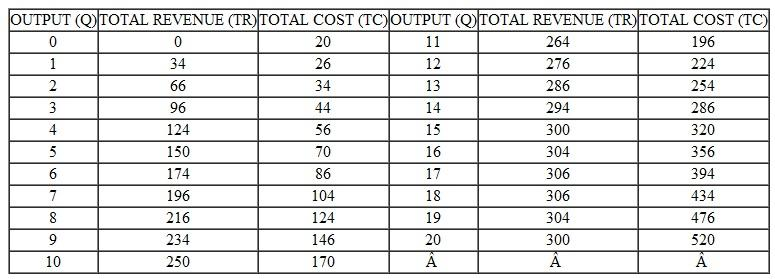a. Compute the marginal revenue and average revenue functions. b. Compute the marginal cost and average cost functions. c. On a single graph, plot the total revenue, total cost, marginal revenue, and marginal cost functions. d. Determine the output level in the graph that maximizes profits (Profit = Total revenue = Total cost) by finding the point where marginal revenue equals marginal cost. e. Check your result in part (d) by finding the output level in the tables developed in parts (a) and (b) that likewise satisfies the condition that marginal revenue equals marginal cost.
Free
Essay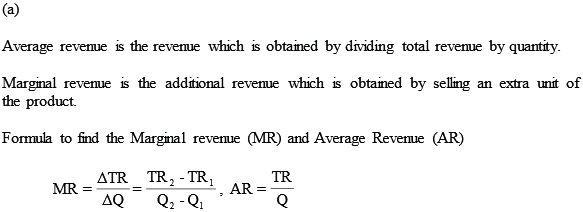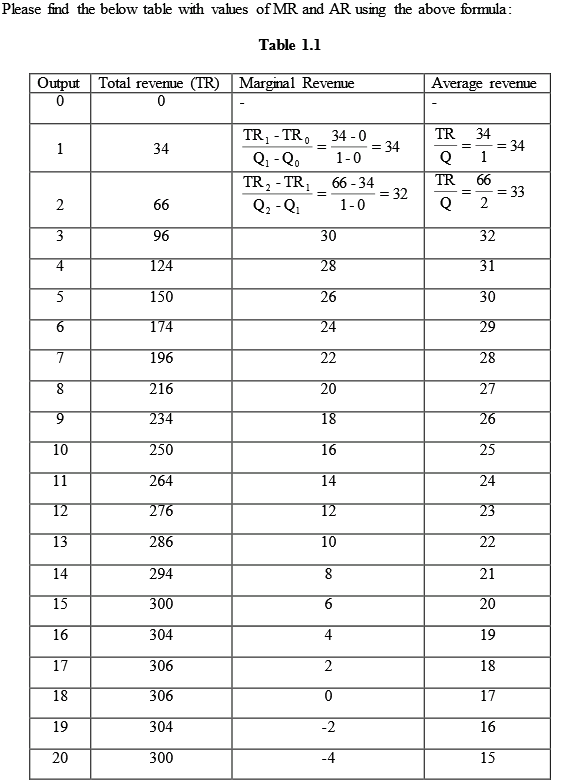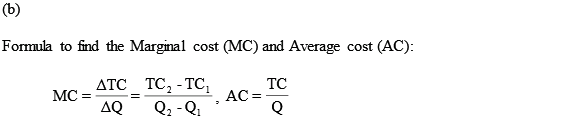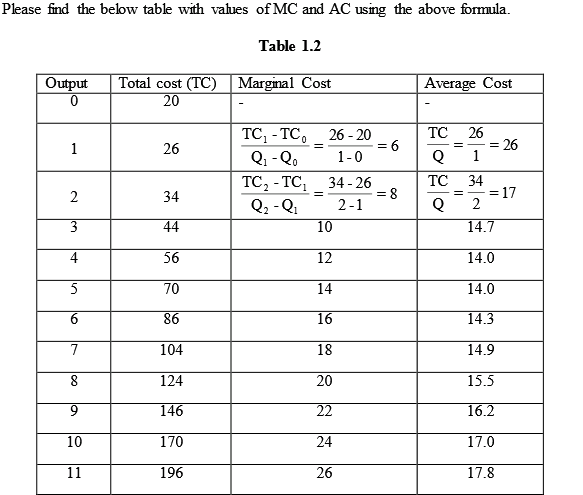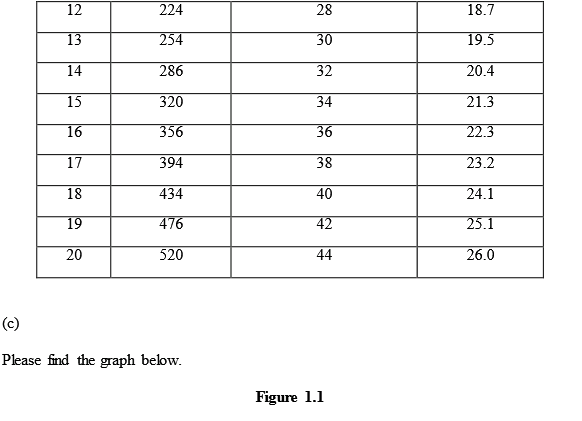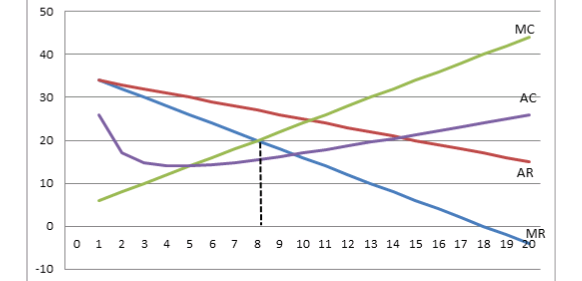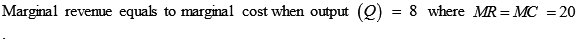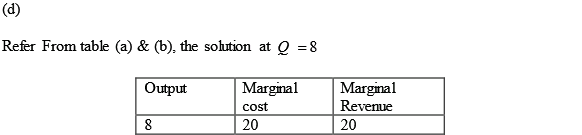TagsConsider again the total revenue and total cost functions shown in tabular form in the previous problem. a. Compute the total, marginal, and average profit functions. b. On a single graph, plot the total profit and marginal profit functions. c. Determine the output level in the graph and table where the total profit function takes on its maximum value. d. How does the result in part (c) in this exercise compare with the result in part (d) of the previous exercise e. Determine total profits at the profit-maximizing output level.
Free
Essay

(a)
Formula to find total profit, marginal profit and average profit: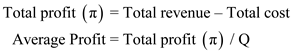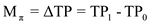Please find the below table with values
Table 1.1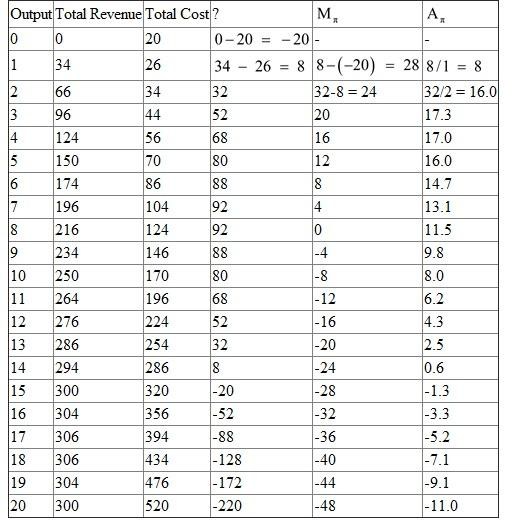(b)
This graph can be plotted manually or using excel.
Graph 1.1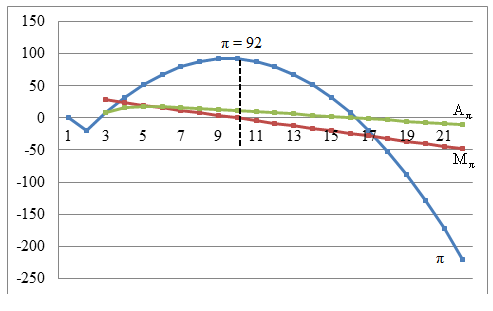(c)
Refer to (b). In the graph, at Q=9, the profit function ( ) is at its maximum.
(d)
Compare to the 1E (d)
The profit maximization is at Q =8 in the previous solution. Here, it is at Q =9. This is only 1 unit variation.
(e)
In the graph plotted in (b), the profit value is 92 at output level of 9.

TagsDifferentiate the following functions: a. TC = 50 + 100Q - 6Q2 +.5Q 3 b. ATC = 50=Q + 100 - 6Q +.5Q 2 c. MC = 100 - 12Q + 1.5Q2 d. Q = 50 -.75P e. Q =.40X 1.50
Free
Essay

(1)
Total cost is shown using the equation.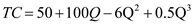Differentiate the equation with respect to Q.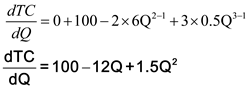(2)
Average Total cost is shown using the equation.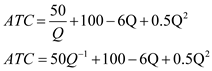Differentiate the equation with respect to Q.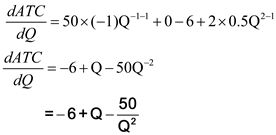(3)
Marginal cost is shown using the equation.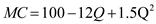Differentiate the equation with respect to Q.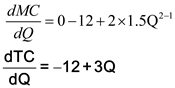(4)
Q is shown using the equation.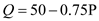Differentiate the equation with respect to P.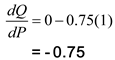(5)
Q is shown using the equation.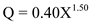Differentiate the equation with respect to X.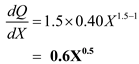TagsDifferentiate the following functions: a. Y = 2X 3 =(4X 2 - 1) b. Y = 2X=(4X 2 - 1) c. Y = 8Z 2 + 4Z - 1, where Z = 2X 2 + 1 (differentiate Y with respect to X)
Essay
TagsDefine Q to be the level of output produced and sold, and assume that the firm's cost function is given by the relationship TC = 20 + 5Q + Q 2 Furthermore, assume that the demand for the output of the firm is a function of price P given by the relationship Q = 25 - P a. Define total profit as the difference between total revenue and total cost, and express in terms of Q the total profit function for the firm. (Note: Total revenue equals price per unit times the number of units sold.) b. Determine the output level where total profits are maximized. c. Calculate total profits and selling price at the profit-maximizing output level. d. If fixed costs increase from $20 to$25 in the total cost relationship, determine the effects of such an increase on the profit-maximizing output level and total profits.
Essay
TagsUse the cost and demand functions in Exercise 5 to calculate the following: a. Determine the marginal revenue and marginal cost functions. b. Show that, at the profit-maximizing output level determined in part (b) of the previous exercise, marginal revenue equals marginal cost and illustrates the economic principle that profits are maximized at the output level where marginal revenue equals marginal cost.
Essay
TagsDetermine the partial derivatives with respect to all of the variables in the following functions: a. TC = 50 + 5Q 1 + 10Q 2 +.5Q 1 Q 2 b. Q = 1.5L.60 K.50 c. Q A = 2.5P A -1.30 Y.20 P B.40
Essay
TagsBounds Inc. determined through regression analysis that its sales (S) are a function of the amount of advertising (measured in units) in two different media. This relationship is given by the following equation (X = newspapers, Y = magazines): S(X,Y) = 200X + 100Y - 10X 2 - 20Y 2 + 20XY a. Find the level of newspaper and magazine advertising that maximizes the firm's sales. b. Calculate the firm's sales at the optimal values of newspaper and magazine advertising determined in part (a).
EssayThe Santa Fe Cookie Factory is considering an expansion of its retail piñon cookie business to other cities. The firm's owners lack the funds needed to undertake the expansion on their own. They are considering a franchise arrangement for the new outlets. The company incurs variable costs of $6 for each pound of cookies sold. The fixed costs of operating a typical retail outlet are estimated to be$300,000 per year. The demand function facing each retail outlet is estimated to be P = $50 -.001Q where P is the price per pound of cookies and Q is the number of pounds of cookies sold. [Note: Total revenue equals price (P) times quantity (Q) sold.] a. What price, output, total revenue, total cost, and total profit level will each profitmaximizing franchise experience b. Assume that the parent company charges each franchisee a fee equal to 5 percent of total revenues, and recompute the values in part (a). c. The Santa Fe Cookie Factory is considering a combined fixed/variable franchise fee structure. Under this arrangement, each franchisee would pay the parent company$25,000 plus 1 percent of total revenues. Recompute the values in part (a). d. What franchise fee arrangement do you recommend that the Santa Fe Cookie Factory adopt What are the advantages and disadvantages of each plan